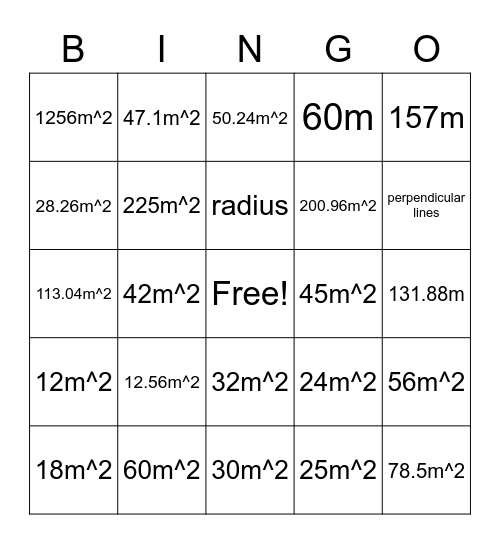# Gr. 7 GeometryThis bingo card has a free space and 42 words: 48m^2, radius, 37.68m, 12.56m^2, 25m^2, 56m^2, 78.5m^2, 16m^2, 50.24m^2, 32m^2, 131.88m, perpendicular lines, 42m^2, 157m, circumference, 24m^2, 36m^2, 113.04m^2, diameter, 47.1m^2, 28.26m^2, 81m^2, parallel lines, 1256m^2, 0.785m^2, 153.86m^2, 200.96m^2, 43.96m, 56.52m, 121m^2, 50m^2, 12m^2, 225m^2, 30m^2, 27m^2, 18m^2, 60m^2, 45m^2, 63m^2, 54m, 60m and 22.85m.

## Play Online